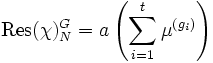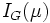# Clifford's theorem

Jump to: navigation, search
This fact is related to: linear representation theory
View other facts related to linear representation theoryView terms related to linear representation theory |

## Statement

### Verbal statement

The restriction of any irreducible complex character of a group, to a normal subgroup, is a multiple of the sum of all conjugates in the whole group of some irreducible character of the normal subgroup.

### Statement with symbols, using character-theoretic language

Let$G$ be a finite group and$N$ a normal subgroup of$G$. Let$\chi$ be a complex irreducible character of$G$ and$\mu$ of$N$ such that:$\langle \operatorname{Res}(\chi)_N^G, \mu \rangle \ne 0$

Then:$\operatorname{Res}(\chi)_N^G = a \left(\sum_{i=1}^t \mu^{(g_i)}\right)$

where$g_i \in G$ and$\mu^{(g)}$ denotes the character:$n \mapsto \mu(gng^{-1})$

Further$a$ and$t$ are positive integers dividing the index$[G:N]$. In fact,$t$ is the index of the subgroup$I_G(\mu)$, defined as:$\left \{ g \in G| \mu^{(g)} = \mu \right \}$$I_G(\mu)$ is termed the inertial subgroup.

Further,$e$ divides the index$[I_G(\mu):N]$.

### Statement with symbols, using module-theoretic language

PLACEHOLDER FOR INFORMATION TO BE FILLED IN: [SHOW MORE]

## Particular cases

### Conjugacy-closed subgroups

A conjugacy-closed subgroup is a subgroup such that any two elements of the subgroup conjugate in the whole group, are also conjugate in the subgroup. If$N$ is conjugacy-closed, then$I_G(\mu) = G$ for any$\mu$ and thus, in this case, the restriction of the irreducible character from$G$ to$N$ is simply a multiple of$\mu$.